CAT  >  CAT Previous Year Questions: Logarithms

# CAT Previous Year Questions: Logarithms - Quantitative Aptitude (Quant)

Question for CAT Previous Year Questions: Logarithms
Try yourself:For a real number a, if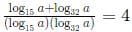then a must lie in the range



*Answer can only contain numeric values
Question for CAT Previous Year Questions: Logarithms
Try yourself:If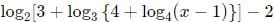= 0 then 4x equals



*Answer can only contain numeric values
Question for CAT Previous Year Questions: Logarithms
Try yourself:If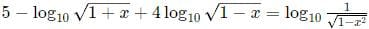,  then 100x equals



*Answer can only contain numeric values
Question for CAT Previous Year Questions: Logarithms
Try yourself:If log4 5 = log4 y log6 √5 , then y equals



Question for CAT Previous Year Questions: Logarithms
Try yourself:If y is a negative number such that 2y2log3 5 = 5log2 3 , then y equals



Question for CAT Previous Year Questions: Logarithms
Try yourself:The value of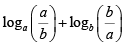, for 1 < a ≤ b cannot be equal to



*Answer can only contain numeric values
Question for CAT Previous Year Questions: Logarithms
Try yourself: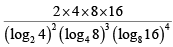equals



Question for CAT Previous Year Questions: Logarithms
Try yourself:In the final examination, Bishnu scored 52% and Asha scored 64%. The marks obtained by Bishnu is 23 less, and that by Asha is 34 more than the marks obtained by Ramesh. The marks obtained by Geeta, who scored 84%, is



Question for CAT Previous Year Questions: Logarithms
Try yourself:Let x and y be positive real numbers such that log5(x + y) + log5(x - y) = 3, and log2y - log2x = 1 - log23. Then xy equals



Question for CAT Previous Year Questions: Logarithms
Try yourself:If x is a real number ,then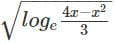is a real number if and only if



Question for CAT Previous Year Questions: Logarithms
Try yourself:The real root of the equation 26x + 23x+2 - 21 = 0 is



Question for CAT Previous Year Questions: Logarithms
Try yourself:If x is a positive quantity such that 2x = 3log52 , then x is equal to



Question for CAT Previous Year Questions: Logarithms
Try yourself:If log2(5 + log3a) = 3 and log5(4a + 12 + log2b) = 3, then a + b is equal to



Question for CAT Previous Year Questions: Logarithms
Try yourself:If log1281 = p, then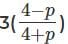is equal to:



Question for CAT Previous Year Questions: Logarithms
Try yourself:If p3 = q4 = r5 = s6, then the value of logs (pqr) is equal to



Question for CAT Previous Year Questions: Logarithms
Try yourself: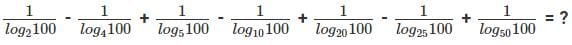Question for CAT Previous Year Questions: Logarithms
Try yourself:Suppose, log3x = log12y = a, where x, y are positive numbers. If G is the geometric mean of x and y, and log6G is equal to:



Question for CAT Previous Year Questions: Logarithms
Try yourself:If 92x – 1 – 81x-1 = 1944, then x is



Question for CAT Previous Year Questions: Logarithms
Try yourself:If x is a real number such that log35 = log5(2 + x), then which of the following is true?



*Answer can only contain numeric values
Question for CAT Previous Year Questions: Logarithms
Try yourself:If log(2a × 3b × 5c) is the arithmetic mean of log(22 × 33 × 5), log(26 × 3 × 57), and log(2 × 32 × 54), then a equals

[TITA 2017]

Question for CAT Previous Year Questions: Logarithms
Try yourself:The value of log0.008√5 + log√381 – 7 is equal to:



Question for CAT Previous Year Questions: Logarithms
Try yourself:If 9x - (1/2) – 22x – 2 = 4x – 32x – 3, then x is



The document CAT Previous Year Questions: Logarithms | Quantitative Aptitude (Quant) is a part of the CAT Course Quantitative Aptitude (Quant).
All you need of CAT at this link: CAT

## Quantitative Aptitude (Quant)

174 videos|144 docs|110 tests

## FAQs on CAT Previous Year Questions: Logarithms - Quantitative Aptitude (Quant)

 1. What is the definition of a logarithm?Ans. A logarithm is the exponent to which a base number must be raised to obtain a given number. It is represented as log base a of b, where a is the base and b is the number.
 2. How do logarithms help in simplifying complex calculations?Ans. Logarithms help in simplifying complex calculations by converting multiplication and division operations into addition and subtraction operations. They also help in solving exponential equations and finding the unknown variable.
 3. How can logarithms be used to solve equations involving exponentials?Ans. Logarithms can be used to solve equations involving exponentials by taking the logarithm of both sides of the equation. This allows us to bring down the exponent as a coefficient and solve for the unknown variable.
 4. What are the properties of logarithms?Ans. The properties of logarithms include the product rule, quotient rule, power rule, and change of base rule. These properties can be used to simplify logarithmic expressions and solve logarithmic equations.
 5. How can logarithms be applied in real-world scenarios?Ans. Logarithms have various applications in real-world scenarios such as population growth, pH calculations, sound intensity measurements, and finance. They help in analyzing exponential growth and decay, as well as in making predictions and comparisons.

## Quantitative Aptitude (Quant)

174 videos|144 docs|110 testsExplore Courses for CAT exam### How to Prepare for CAT

Read our guide to prepare for CAT which is created by Toppers & the best Teachers
Signup to see your scores go up within 7 days! Learn & Practice with 1000+ FREE Notes, Videos & Tests.
10M+ students study on EduRev
Track your progress, build streaks, highlight & save important lessons and more!
Related Searches

,

,

,

,

,

,

,

,

,

,

,

,

,

,

,

,

,

,

,

,

,

;